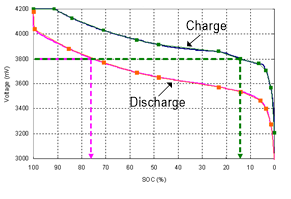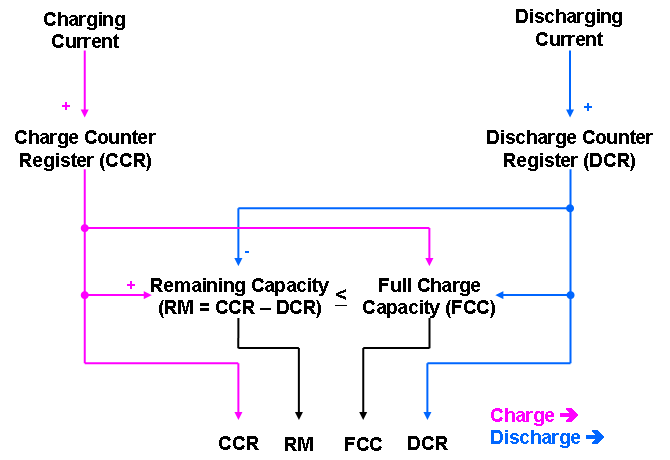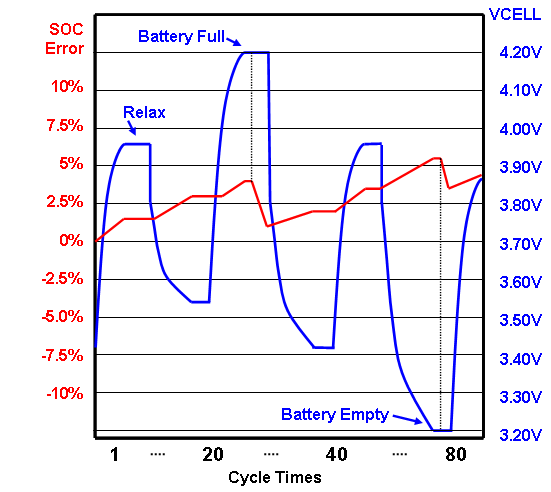# The theory of Lithium charge and discharge & the design of electricity calculation method(2)

The theory of Lithium charge and discharge & the design of electricity calculation method

2. Introduction to battery meter

2.1 Function introduction of electricity meter

Battery management can be considered as part of power management. In battery management, the electricity meter is responsible for estimating the battery capacity. Its basic function is to monitor the voltage, charge/discharge current and battery temperature, and estimate the state of charge (SOC) and the full charge capacity (FCC) of the battery. There are two typical methods to estimate the state of charge of battery: open-circuit voltage method (OCV) and coulometric method. The other method is the dynamic voltage algorithm designed by RICHTEK.

2.2 Open circuit voltage method

It is easy to realize the electricity meter using the open-circuit voltage method, which can be obtained by checking the corresponding state of charge of the open-circuit voltage. The open circuit voltage is assumed to be the battery terminal voltage when the battery is resting for more than 30 minutes.

The battery voltage curve will vary with different load, temperature and battery aging. Therefore, a fixed open-circuit voltmeter cannot fully represent the state of charge; State of charge cannot be estimated by looking up the table alone. In other words, if the state of charge is estimated only by looking up the table, the error will be large.

The figure below shows that the state of charge (SOC) of the same battery voltage is very different by the open-circuit voltage method under charging and discharging.Figure 5. Battery voltage under charging and discharging conditions

It can be seen from the figure below that the state of charge varies greatly under different loads during discharge. So basically, the open-circuit voltage method is only suitable for systems that require low accuracy of the state of charge, such as cars using lead-acid batteries or uninterruptible power supplies.Figure 6. Battery voltage under different loads during discharge

2.3 Coulometric method

The operating principle of coulometry is to connect a detection resistor on the charging/discharging path of the battery. ADC measures the voltage on the detection resistance and converts it into the current value of the battery being charged or discharged. The real-time counter (RTC) can integrate the current value with time to know how many coulombs are flowing.Figure 7. Basic working mode of coulomb measurement method

Coulometric method can accurately calculate the real-time state of charge during charging or discharging. With the charge coulomb counter and discharge coulomb counter, it can calculate the residual electric capacity (RM) and the full charge capacity (FCC). At the same time, the remaining charge capacity (RM) and the full charge capacity (FCC) can also be used to calculate the state of charge (SOC=RM/FCC). In addition, it can also estimate the remaining time, such as power exhaustion (TTE) and power fullness (TTF).Figure 8. Calculation formula of coulomb method

There are two main factors that cause the accuracy deviation of coulomb metrology. The first is the accumulation of offset error in current sensing and ADC measurement. Although the measurement error is relatively small with the current technology, if there is no good method to eliminate it, the error will increase with time. The figure below shows that in practical application, if there is no correction in the time duration, the accumulated error is unlimited.Figure 9. Cumulative error of coulomb method

In order to eliminate the accumulated error, there are three possible time points in normal battery operation: end of charge (EOC), end of discharge (EOD) and rest (Relax). The battery is fully charged and the state of charge (SOC) should be 100% when the charging end condition is reached. The discharge end condition means that the battery has been completely discharged and the state of charge (SOC) should be 0%; It can be an absolute voltage value or change with the load. When reaching the rest state, the battery is neither charged nor discharged, and it remains in this state for a long time. If the user wants to use the rest state of the battery to correct the error of the coulometric method, he must use an open-circuit voltmeter at this time. The figure below shows that the state of charge error under the above conditions can be corrected.Figure 10. Conditions for eliminating the cumulative error of coulometric method

The second main factor causing the accuracy deviation of coulomb metering method is the full charge capacity (FCC) error, which is the difference between the design capacity of the battery and the real full charge capacity of the battery. Full charge capacity (FCC) will be affected by temperature, aging, load and other factors. Therefore, the re-learning and compensation method of fully charged capacity is very important for coulometric method. The figure below shows the trend of SOC error when the full charge capacity is overestimated and underestimated.Figure 11. Error trend when full charge capacity is overestimated and underestimated

Post time: Feb-15-2023
•#### Phone

•